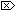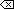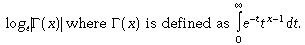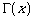The Open Group Base Specifications Issue 6
IEEE Std 1003.1-2001

#### NAME

lgamma, lgammaf, lgammal - log gamma function

#### SYNOPSIS

```#include <math.h> double lgamma(double x); float lgammaf(float x); long double lgammal(long double x); ```

`[XSI]extern int signgam;`

#### DESCRIPTION

[CX]The functionality described on this reference page is aligned with the ISO C standard. Any conflict between the requirements described here and the ISO C standard is unintentional. This volume of IEEE Std 1003.1-2001 defers to the ISO C standard.These functions shall computeThe argument x need not be a non-positive integer (is defined over the reals, except the non-positive integers).

[XSI]The sign ofis returned in the external integer signgam.[CX]These functions need not be reentrant. A function that is not required to be reentrant is not required to be thread-safe.An application wishing to check for error situations should set errno to zero and call feclearexcept(FE_ALL_EXCEPT) before calling these functions. On return, if errno is non-zero or fetestexcept(FE_INVALID | FE_DIVBYZERO | FE_OVERFLOW | FE_UNDERFLOW) is non-zero, an error has occurred.

#### RETURN VALUE

Upon successful completion, these functions shall return the logarithmic gamma of x.

If x is a non-positive integer, a pole error shall occur and lgamma(), lgammaf(), and lgammal() shall return +HUGE_VAL, +HUGE_VALF, and +HUGE_VALL, respectively.

If the correct value would cause overflow, a range error shall occur and lgamma(), lgammaf(), and lgammal() shall return ±HUGE_VAL, ±HUGE_VALF, and ±HUGE_VALL (having the same sign as the correct value), respectively.

[MX]If x is NaN, a NaN shall be returned.

If x is 1 or 2, +0 shall be returned.

If x is ±Inf, +Inf shall be returned.#### ERRORS

These functions shall fail if:

Pole Error
The x argument is a negative integer or zero.

If the integer expression (math_errhandling & MATH_ERRNO) is non-zero, then errno shall be set to [ERANGE]. If the integer expression (math_errhandling & MATH_ERREXCEPT) is non-zero, then the divide-by-zero floating-point exception shall be raised.

Range Error
The result overflows.

If the integer expression (math_errhandling & MATH_ERRNO) is non-zero, then errno shall be set to [ERANGE]. If the integer expression (math_errhandling & MATH_ERREXCEPT) is non-zero, then the overflow floating-point exception shall be raised.

The following sections are informative.

None.

#### APPLICATION USAGE

On error, the expressions (math_errhandling & MATH_ERRNO) and (math_errhandling & MATH_ERREXCEPT) are independent of each other, but at least one of them must be non-zero.

None.

#### FUTURE DIRECTIONS

None.

exp() , feclearexcept() , fetestexcept() , isnan() , the Base Definitions volume of IEEE Std 1003.1-2001, Section 4.18, Treatment of Error Conditions for Mathematical Functions, <math.h>

#### CHANGE HISTORY

First released in Issue 3.

#### Issue 5

The DESCRIPTION is updated to indicate how an application should check for an error. This text was previously published in the APPLICATION USAGE section.

A note indicating that this function need not be reentrant is added to the DESCRIPTION.

#### Issue 6

The lgamma() function is no longer marked as an extension.

The lgammaf() and lgammal() functions are added for alignment with the ISO/IEC 9899:1999 standard.

The DESCRIPTION, RETURN VALUE, ERRORS, and APPLICATION USAGE sections are revised to align with the ISO/IEC 9899:1999 standard.

IEC 60559:1989 standard floating-point extensions over the ISO/IEC 9899:1999 standard are marked.

XSI extensions are marked.

End of informative text.

UNIX ® is a registered Trademark of The Open Group.
POSIX ® is a registered Trademark of The IEEE.
[ Main Index | XBD | XCU | XSH | XRAT ]